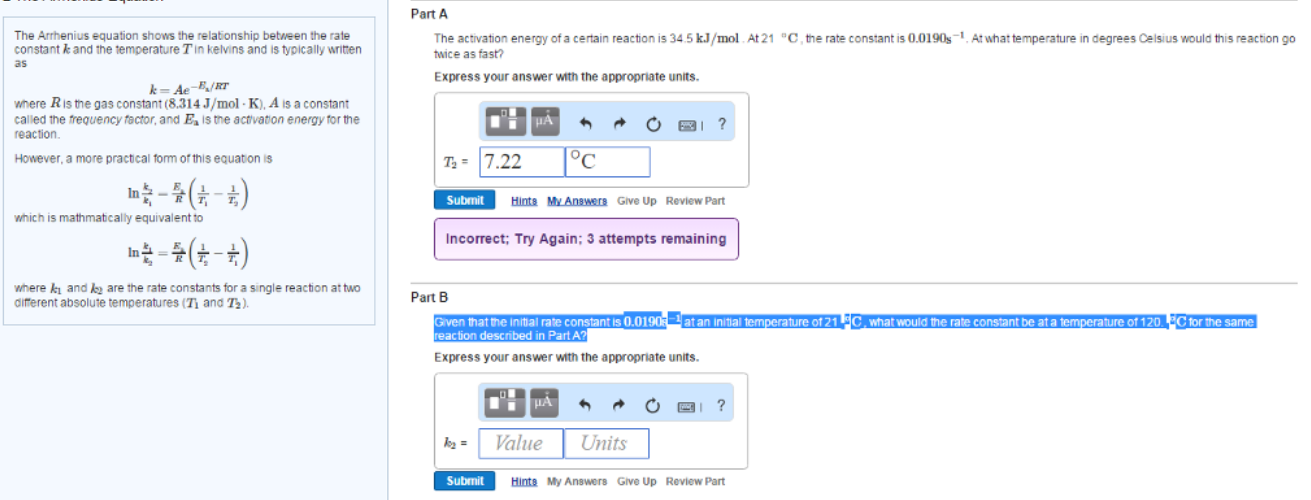# Problem: The activation energy of a certain reaction is 34.5 Kj/mol. At 21°C, the rate constant is 0.0190s-1  . At what temperature at degrees Celsius would this reaction go twice as fast.Given the initial rate constant is 0.0190s-1 at an initial temperature of 21°C what would the rate constant be at a temperature of 120 °C for the same reaction described in Part A?

###### FREE Expert Solution
94% (104 ratings)###### Problem Details

The activation energy of a certain reaction is 34.5 Kj/mol. At 21°C, the rate constant is 0.0190s-1  . At what temperature at degrees Celsius would this reaction go twice as fast.

Given the initial rate constant is 0.0190s-1 at an initial temperature of 21°C what would the rate constant be at a temperature of 120 °C for the same reaction described in Part A?